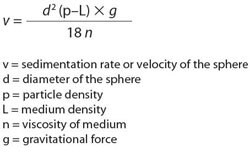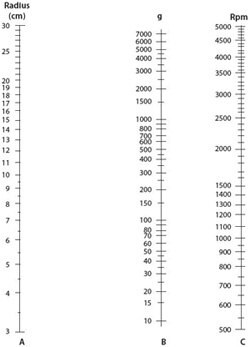HomeProtein Pull-DownCentrifugation Basics

# Centrifugation Basics

## Natural Gravitational Forces and Centrifugation

The earth's gravitational force is sufficient to separate many types of particles over time. A tube of anticoagulated whole blood left standing on a bench top will eventually separate into plasma, red blood cells, and white blood cell fractions. However, the length of time required precludes this manner of separation for most applications.

In practice, centrifugal force is necessary to separate most particles. In addition, the potential degradation of biological compounds during prolonged storage means faster separation techniques are needed.

Centrifugation is one of the most basic of laboratory applications and is used by a wide range of clinical and research personnel. Essentially, centrifugation is the separation of particles by sedimentation. Even though sedimentation using centrifugation is not a new technology, it is essential for cutting-edge genomic and proteomic research by providing purified particles of interest.

The rate of separation in a suspension of particles by way of gravitational force mainly depends on the particle size and density. Particles of higher density or larger size typically travel at a faster rate and at some point will be separated from particles less dense or smaller. This sedimentation of particles, including cells, can be explained by Stoke's law, which describes the movement of a sphere in a gravitational field.1 The equation calculates the velocity of sedimentation utilizing five parameters (Figure 1).Figure 1.The Stoke's Law equation.

## Particle Behavior and Separation

From the Stokes equation five important behaviors of particles can be explained:

1. The rate of particle sedimentation is proportional to the particle size.
2. The sedimentation rate is proportional to the difference in density between the particle and the medium.
3. The sedimentation rate is zero when the particle density is the same as the medium density.
4. The sedimentation rate decreases as the medium viscosity increases.
5. The sedimentation rate increases as the gravitational force increases.

## Relative Centrifugal Force

Most particles are so small that gravitational force is insufficient to overcome the random molecular forces of particles to influence separation. Centrifugation, the name given to separation applications which involve spinning around an axis to produce a centrifugal force, is a way to increase the magnitude of the gravitational field. The particles in suspension experience a radial centrifugal force moving them away from the axis of rotation.2

The radial force generated by the spinning rotor is expressed relative to the earth's gravitational force and therefore is known as the relative centrifugal force (RCF) or the "g force." The g force acting on particles is exponential to the speed of rotation (defined as revolutions per minute; rpm).

Doubling the speed of rotation increases the centrifugal force by a factor of four. The centrifugal force also increases with the distance from the axis of rotation. These two parameters are of considerable significance when selecting the appropriate centrifuge. Table 1 summarizes the applications that can be classified by the relative centrifugal force.3

Table 1Classes of centrifuges and their applications

* Can be done but not usually used for this purpose.

RCF is dependent on the speed of rotation in rpm and the distance of the particles from the center of rotation. When the speed of rotation is given in rpm (Q) and the distance (r) is expressed in centimeters, RCF can be calculated by using the formula in Figure 2.Figure 2.Formula for relative centrifugal force (RCF).

## Low-Speed Centrifugation Nomogram

A nomogram can also be used to obtain the speed of a centrifuge rotor necessary for a desired RCF (Figure 3). This quick estimate is useful for low speed centrifugation applications. However, it is more accurate to use the RCF calculation for speeds in excess of 10,000 rpm.Figure 3.Nomogram for estimation of centrifuge rpm setting.

## Nomogram Instructions

1. Measure the radius (cm) from the center of the centrifuge rotor to the end of test tube carrier.
2. Obtain the relative centrifugal force necessary for the application.
3. A straight line connecting the value of the radius with the relative centrifugal force (g) value will enable the speed of the rotor (rpm) to be read off of the right column.

Density gradient centrifugation is a technique that allows the separation of particles on the basis of their size, shape, and density. A density gradient is typically created by layering media of increasing density in a centrifuge tube. When a sample is layered on top of a density gradient and centrifuged, the various particles move through the gradient at different rates. The particles appear as bands or zones in the gradient with the more dense and larger particles migrating furthest.

A number of different compounds have been investigated as density gradient media. One of the first density gradient centrifugation techniques was developed in the 1950s and used a buffered sucrose solution for the purification of cell organelles. Sucrose quickly became the density medium of choice for separating homogenized mammalian tissues. Later, cesium chloride gradients were used to separate DNA of different densities. Meselson and Stahl in 1958 used cesium chloride density gradient centrifugation in an elegant experiment to support the semi-conservative model of DNA replication. Colloidal-silica suspensions were first manufactured by DuPont and sold under the name of LUDOX®.4

In 1977, the stabilized silica colloid coated with polyvinylpyrrolidone (PVP) called Percoll® became available for separating cells and subcellular particles. In 1968, Boyum described methods for the isolation of mononuclear cells from circulating blood and bone marrow using mixtures of polysaccharide and a radiopaque contrast medium. This led to the development of the first nonionic iodinated density gradient medium, metrizamide, in the 1970s.5 Now, a large selection of commercial iodinated density gradient media are available.

### References

1.
Sharpe PT. 2012. Methods of Cell Separation. Elsevier Science.
2.
Graham J. 2001. Biological Centrifugation. 1. Garland Science.
3.
Rickwood D, Ford T, Steensgaard J. 1994. Centrifugation: Essential Data. Wiley.
4.
Rickwood D. 1984. Centrifugation: A Practical Approach. 2. Oxford University Press.
5.
Rickwood D. 1983. Iodinated Density Gradient Media, A Practical Approach. Oxford University Press.